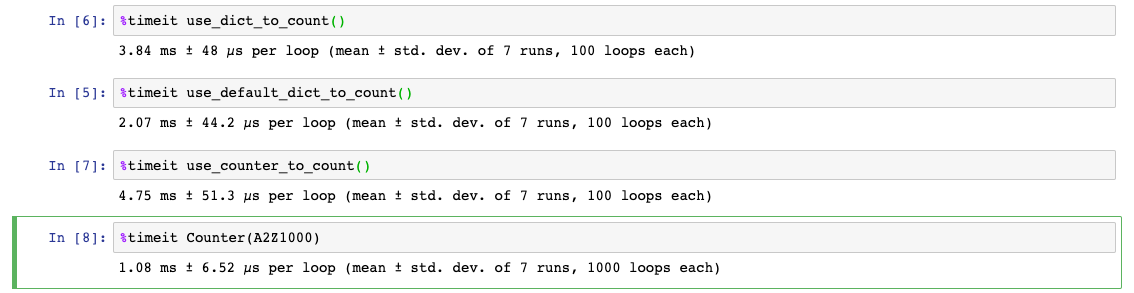Python collections 模組提供多個方便的類別(class)供開發者利用，其中 Counter 類別（dict 的子類別）可以應用在計數(counting)相關的場景：

A Counter is a dict subclass for counting hashable objects.

• Python 3.7

### Counter 類別介紹

``````counters = {
"words": 123,
"stopwords": 4,
"sentences": 6,
}``````

``````if key in counters:
counters[key] += 1
else:
counters[key] = 1``````

``````counters.setdefault(key, 0)
counters[key] += 1``````

Counter 是在 Python 3.1 之後加入的新類別(class), 可以說集前述做法於一身，並進一步簡化的成果。使用 Counter 後，開發者們不需再檢查 key 是否存在字典中，也不需為 key 值設定預設值（直接預設為 0），因此使用上可以直接簡化為：

``````from collections import Counter

counters = Counter(
{
'words': 123,
'stopwords': 4,
'sentences': 6,
}
)

counters['words'] += 1
counters['new item'] += 1``````

``````from collections import Counter

counters = Counter(
{
'words': 123,
'stopwords': 4,
'sentences': 6,
}
)

for k, v in counters.items():
print(k, v)``````

Counter 類別也有內建一些實用的方法，像 most_common{} 可以幫助我們取的前 n 大的 key 與 value, 並且遞減排序，或者 [例如以下範例取得 counters 中的前 3 大 key 與 value:

``````>>> counters.most_common(3)  # TOP 3
[('words', 123), ('sentences', 6), ('stopwords', 4)]``````

Counter 類別接受帶入參數作為初始值，參數可以是 iterable, dict 甚至是 keyword arguments, 例如以下範例，其執行結果都是相同的：

``````counters = Counters({'a': 1, 'b': 2})

counters = Counter(['a', 'b', 'b'])

counters = Counters('abb')

counters = Counters(a=1, b=2)``````

Counter 也支援 `+` `-` 運算，加法會將 2 個 Counter 中所有的 key 合併，並對相同 key 的值進行加總：

``````>>> Counter(a=1, b=1) + Counter(a=1, b=1, c=1)
Counter({'a': 2, 'b': 2, 'c': 1})``````

``````>>> Counter(a=3, b=1) - Counter(a=1, b=1, c=1)
Counter({'a': 2})``````

``````>>> Counter('abbb') | Counter('bcc')
Counter({'b': 3, 'c': 2, 'a': 1})``````

``````>>> Counter('abbbc') & Counter('bcc')
Counter({'b': 1, 'c': 1})``````

### 執行效率比較(Benchmark)

``````from collections import Counter, defaultdict

A2Z1000 = ''.join(chr(i) for i in range(97, 123)) * 1000

def use_dict_to_count():
d = dict()
for c in A2Z1000:
d.setdefault(c, 0)
d[c] += 1

def use_default_dict_to_count():
d = defaultdict(int)
for c in A2Z1000:
d[c] += 1

def use_counter_to_count():
d = Counter()
for c in A2Z1000:
d[c] += 1````````````def use_counter_to_count():
d = Counter()
d.update(A2Z1000)``````

Happy Coding!

https://github.com/python/cpython/blob/7acedd71dec4e60400c865911e8961dbb49d5597/Lib/collections/__init__.py#L534

https://docs.python.org/3/library/collections.html#collections.Counter